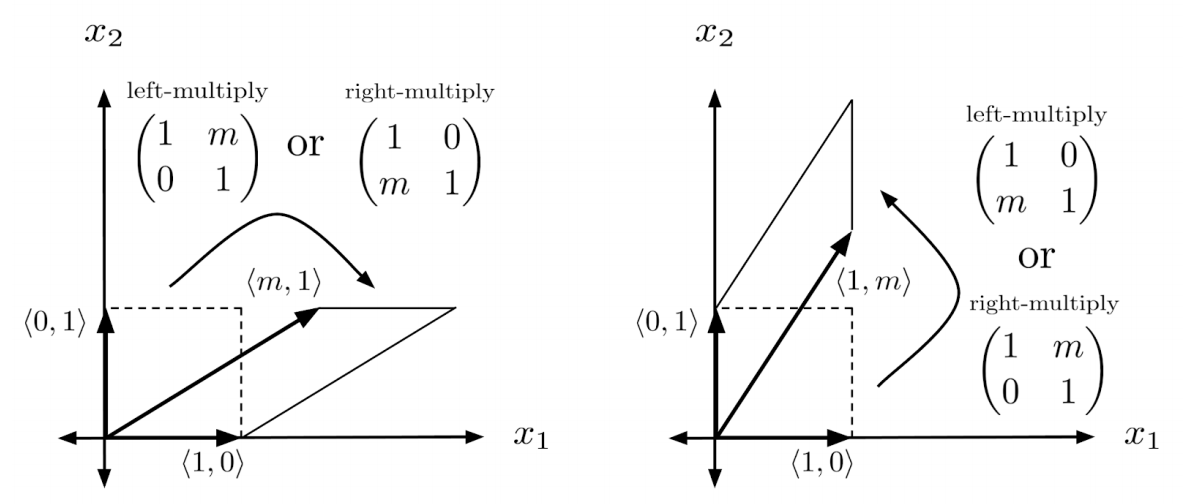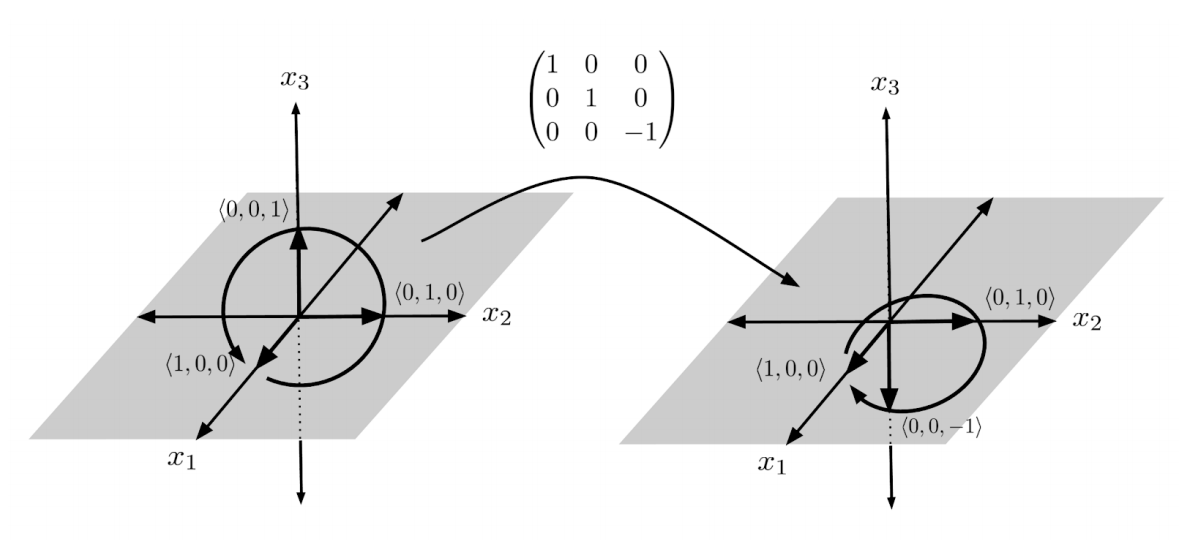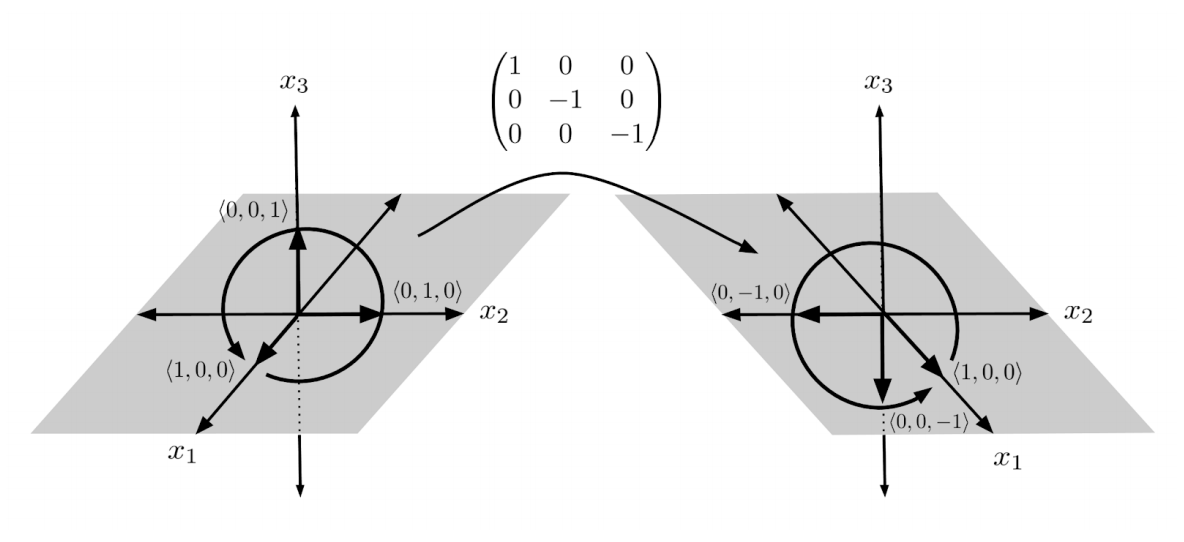# Rescaling, Shearing, and the Determinant

This post is part of a series.

The key insight in this chapter is that every square matrix can be decomposed into a product of rescalings and shears. Before we elaborate on that, though, let’s discuss what rescalings and shears are, in terms of matrices.

## Rescaling Matrices

Rescaling matrices are matrices that rescale the dimensions of space, with each dimension potentially being rescaled by a different amount. That is to say, the dimensions of space maintain their original direction, but their lengths are multiplied by some factors.

For example, in 2-dimensional space, the rescaling of $\left< 1,0 \right>$ into $\left< 2,0 \right>$ and $\left< 0, \frac{1}{3} \right>$ into is given by left- or right-multiplication by the following rescaling matrix:

\begin{align*} \begin{pmatrix} 2 & 0 \\ 0 & \frac{1}{3} \end{pmatrix} \end{align*}

We could also have negative rescalings, or even zero rescalings that collapse a vector’s length down to $0$. For example, the rescaling matrix that rescales $\left< 1,0 \right>$ into $\left< -5,0 \right>$ and $\left< 0,1 \right>$ into $\left< 0,0 \right>$ is given by

\begin{align*} \begin{pmatrix} -5 & 0 \\ 0 & 0 \end{pmatrix}. \end{align*}

We can extend to higher dimensions as well. In 3-dimensional space, the rescaling matrix that rescales $\left< 1,0,0 \right>$ to $\left< 2,0,0 \right>$, and $\left< 0,1,0 \right>$ to $\left< 0,-3,0 \right>$, and $\left< 0,0,1 \right>$ to $\left< 0,0,\frac{4}{5} \right>$ is given by

\begin{align*} \begin{pmatrix} 2 & 0 & 0 \\ 0 & -3 & 0 \\ 0 & 0 & \frac{4}{5} \end{pmatrix}. \end{align*}

Do you notice a pattern? Rescaling matrices are just diagonal matrices!

There is a fast trick for multiplying rescaling matrices: just multiply the diagonal entries independently. Consequently, the product of two rescaling matrices is itself always a rescaling matrix as well.

\begin{align*} \begin{pmatrix} 2 & 0 & 0 \\ 0 & -3 & 0 \\ 0 & 0 & \frac{4}{5} \end{pmatrix} \begin{pmatrix} 4 & 0 & 0 \\ 0 & \frac{1}{2} & 0 \\ 0 & 0 & 5 \end{pmatrix} =\begin{pmatrix} (2)(4) & 0 & 0 \\ 0 & \left( -3 \right) \left( \frac{1}{2} \right) & 0 \\ 0 & 0 & \left( \frac{4}{5} \right) \left( 5 \right) \end{pmatrix} =\begin{pmatrix} 8 & 0 & 0 \\ 0 & -\frac{3}{2} & 0 \\ 0 & 0 & 4 \end{pmatrix} \end{align*}

Likewise, there is also a fast trick to compute the determinant of a rescaling matrix. Since the vectors in a rescaling matrix form a rectangular prism, and the volume of that prism is obtained by multiplying the side lengths, the determinant of a rescaling matrix is simply the product of the rescalings, i.e. the product of the diagonal.

\begin{align*} \mbox{det} \begin{pmatrix} 8 & 0 & 0 \\ 0 & -\frac{3}{2} & 0 \\ 0 & 0 & 4 \end{pmatrix} = \left( 8 \right) \left( -\frac{3}{2} \right) \left( 4 \right) = -48 \end{align*}

## Shearing Matrices

Now let’s talk about shearing matrices. Recall that shearing involves moving one of the sides of a parallelepiped in a parallel direction, and does not change the volume of the parallelepiped. We have also seen that in a set of vectors, shearing simply amounts to adding a multiple of some vector to a different vector.

Since a matrix is defined by its transformation of the unit cube, we can consider just the shears of the unit cube. In 2 dimensions, for example, a shear of the unit cube would either consist of vectors $\left< 1,0 \right>$ and $\left< m,1 \right>$, or $\left< 1,m \right>$ and $\left< 0,1 \right>$, where $m$ is the multiple of the vector that is added.Likewise, in 3 dimensions, a shear of the unit cube could consist of vectors $\left< 1,0,0 \right>$, $\left< 0,1,0 \right>$, and $\left< m,n,1 \right>$; or $\left< 1,0,0 \right>$, $\left< m,1,n \right>$, and $\left< 0,0,1 \right>$; or $\left< 1,0,0 \right>$, $\left< 0,1,0 \right>$, and $\left< m,n,1 \right>$, where $m$ and $n$ are the multiples of the vectors that are added. These correspond to the following matrices, respectively:

\begin{align*} \underline{\text{Left-multiply}} &\hspace{.5cm} \begin{pmatrix} 1 & 0 & m \\ 0 & 1 & n \\ 0 & 0 & 1 \end{pmatrix} \hspace{.5cm} \begin{pmatrix} 1 & m & 0 \\ 0 & 1 & 0 \\ 0 & n & 1 \end{pmatrix} \hspace{.5cm} \begin{pmatrix} 1 & 0 & 0 \\ m & 1 & 0 \\ n & 0 & 1 \end{pmatrix} \\ \text{OR} \hspace{1cm}& \\ \underline{\text{Right-multiply}} &\hspace{.5cm} \begin{pmatrix} 1 & 0 & 0 \\ 0 & 1 & 0 \\ m & n & 1 \end{pmatrix} \hspace{.5cm} \begin{pmatrix} 1 & 0 & 0 \\ m & 1 & n \\ 0 & 0 & 1 \end{pmatrix} \hspace{.5cm} \begin{pmatrix} 1 & m & n \\ 0 & 1 & 0 \\ 0 & 0 & 1 \end{pmatrix} \\ \end{align*}

Do you notice a pattern? Shear matrices consist of a diagonal of 1s, with all other entries zero except for possibly a single row or column.

Unfortunately, there is no easy trick for multiplying shear matrices, other than just adding multiples of one row/column to another row/column. The result of multiplying two shear matrices might not even maintain a diagonal of 1s, for example:

\begin{align*} \begin{pmatrix} 1 & a \\ 0 & 1 \end{pmatrix} \begin{pmatrix} 1 & 0 \\ b & 1 \end{pmatrix} = \begin{pmatrix} 1+ab & a \\ b & 1 \end{pmatrix} \end{align*}

However, there is one property that is conserved in the result of multiplying shear matrices: the determinant of a product of shear matrices has to remain 1. This is because shear matrices don’t change the volume of any parallelepiped within a vector space.

## Decomposing into Rescalings and Shears

Now let’s move onto the main idea of this chapter: every square matrix can be decomposed into a product of rescalings and shears. We’ll illustrate the process through a couple of examples.

The process of decomposing a matrix into a product of rescalings and shears is very familiar – it mainly consists of reducing the row or column vectors while keeping track of our multipliers in rescaling and shear vectors.

The only catch is that we need to keep track of the process in reverse, which means we have to flip the sign of the multipliers that we put in shear matrices, and take the reciprocal of the multipliers that we put in rescaling matrices.

For example, to decompose the matrix below into a product of rescalings and shears, we start by adding $-3$ times the first row to the second row, which means we put $3$ in our left shear matrix to represent the reverse operation. Then, we multiply the bottom row by $-\frac{1}{2}$, which means we put $-2$ in our left rescaling matrix to represent the reverse operation.

\begin{align*} &\begin{pmatrix} 1 & 2 \\ 3 & 4 \end{pmatrix} \\ &\begin{pmatrix} 1 & 0 \\ 3 & 1 \end{pmatrix} \begin{pmatrix} 1 & 2 \\ 0 & -2 \end{pmatrix} \\ &\begin{pmatrix} 1 & 0 \\ 3 & 1 \end{pmatrix} \begin{pmatrix} 1 & 0 \\ 0 & -2 \end{pmatrix} \begin{pmatrix} 1 & 2 \\ 0 & 1 \end{pmatrix} \end{align*}

Here is another example, which might initially seem tricky because the first row has a $0$ as its first entry. However, we can create a $1$ as the first entry by adding $-\frac{5}{4}$ of the second row. Then, all that remains is to rescale the second row.

\begin{align*} &\begin{pmatrix} 0 & 3 \\ -\frac{4}{5} & 0 \end{pmatrix} \\ &\begin{pmatrix} 1 & \frac{5}{4} \\ 0 & 1 \end{pmatrix} \begin{pmatrix} 1 & 3 \\ -\frac{4}{5} & 0 \end{pmatrix} \\ &\begin{pmatrix} 1 & \frac{5}{4} \\ 0 & 1 \end{pmatrix} \begin{pmatrix} 1 & 0 \\ 0 & -\frac{4}{5} \end{pmatrix} \begin{pmatrix} 1 & 3 \\ 1 & 0 \end{pmatrix} \end{align*}

Note that sometimes we may need to rescale by $0$ to introduce a $1$ into a row of $0$s, such as in the top row of the matrix below.

\begin{align*} \begin{pmatrix} 0 & 0 \\ 1 & 2 \end{pmatrix} = \begin{pmatrix} 0 & 0 \\ 0 & 2 \end{pmatrix} \begin{pmatrix} 1 & 0 \\ \frac{1}{2} & 1 \end{pmatrix} \end{align*}

Likewise, to introduce a $1$ into a column of $0$s, we can right-multiply by a rescaling matrix having a $0$ entry on the diagonal.

\begin{align*} \begin{pmatrix} 0 & 1 \\ 0 & 2 \end{pmatrix} = \begin{pmatrix} 1 & \frac{1}{2} \\ 0 & 1 \end{pmatrix} \begin{pmatrix} 0 & 0 \\ 0 & 2 \end{pmatrix} \end{align*}

Below is a final example of decomposing a $3 \times 3$ matrix into rescalings and shears.

\begin{align*} &\begin{pmatrix}0 & 2 & 0 \\ 2 & 3 & 0 \\ 0 & 4 & 5 \end{pmatrix} \\ &\begin{pmatrix} 1 & \frac{2}{3} & 0 \\ 0 & 1 & 0 \\ 0 & 0 & 1 \end{pmatrix} \begin{pmatrix} -\frac{4}{3} & 0 & 0 \\ 2 & 3 & 0 \\0 & 4 & 5 \end{pmatrix} \\ & \begin{pmatrix} 1 & \frac{2}{3} & 0 \\ 0 & 1 & 0 \\ 0 & 0 & 1 \end{pmatrix} \begin{pmatrix} -\frac{4}{3} & 0 & 0 \\ 0 & 1 & 0 \\ 0 & 0 & 1 \end{pmatrix} \begin{pmatrix} 1 & 0 & 0 \\ 2 & 3 & 0 \\0 & 4 & 5 \end{pmatrix} \\ &\begin{pmatrix} 1 & \frac{2}{3} & 0 \\ 0 & 1 & 0 \\ 0 & 0 & 1 \end{pmatrix} \begin{pmatrix} -\frac{4}{3} & 0 & 0 \\ 0 & 1 & 0 \\ 0 & 0 & 1 \end{pmatrix} \begin{pmatrix} 1 & 0 & 0 \\ 2 & 1 & 0 \\ 0 & 0 & 1 \end{pmatrix} \begin{pmatrix} 1 & 0 & 0 \\ 0 & 3 & 0 \\0 & 4 & 5 \end{pmatrix} \\ & \begin{pmatrix} 1 & \frac{2}{3} & 0 \\ 0 & 1 & 0 \\ 0 & 0 & 1 \end{pmatrix} \begin{pmatrix} -\frac{4}{3} & 0 & 0 \\ 0 & 1 & 0 \\ 0 & 0 & 1 \end{pmatrix} \begin{pmatrix} 1 & 0 & 0 \\ 2 & 1 & 0 \\ 0 & 0 & 1 \end{pmatrix} \begin{pmatrix} 1 & 0 & 0 \\ 0 & 3 & 0 \\ 0 & 0 & 1 \end{pmatrix} \begin{pmatrix} 1 & 0 & 0 \\ 0 & 1 & 0 \\0 & 4 & 5 \end{pmatrix} \\ &\begin{pmatrix} 1 & \frac{2}{3} & 0 \\ 0 & 1 & 0 \\ 0 & 0 & 1 \end{pmatrix} \begin{pmatrix} -\frac{4}{3} & 0 & 0 \\ 0 & 1 & 0 \\ 0 & 0 & 1 \end{pmatrix} \begin{pmatrix} 1 & 0 & 0 \\ 2 & 1 & 0 \\ 0 & 0 & 1 \end{pmatrix} \begin{pmatrix} 1 & 0 & 0 \\ 0 & 3 & 0 \\ 0 & 0 & 1 \end{pmatrix} \begin{pmatrix} 1 & 0 & 0 \\ 0 & 1 & 0 \\ 0 & 4 & 1 \end{pmatrix} \begin{pmatrix} 1 & 0 & 0 \\ 0 & 1 & 0 \\0 & 0 & 5 \end{pmatrix} \end{align*}

## Determinant of a Product

One important consequence of decomposing square matrices into rescalings and shears is that, for two square matrices $A$ and $B$, we have

\begin{align*} \det(AB) = \det(A)\det(B). \end{align*}

To understand why this is, imagine writing $A$ and $B$ each as a product of rescalings and shears.

Since the shears have no effect on volume, they can be removed from the product $AB$ without changing $\det(AB)$.

Then, we are left with the rescaling matrices for $A$ and $B$, which give the determinants for $A$ and $B$, respectively.

## Meaning of Negative Determinant

Another consequence of decomposing square matrices into rescalings and shears is that it makes clear the meaning of negative determinant.

Since shears don’t change the determinant, a negative in a determinant must come from the rescalings – meaning that the total number of negative entries in the diagonals of all the rescaling matrices must be odd.

There is geometric intuition for negative determinants as well, having to do with the orientation of space.

The orientation of space can be thought of as a “curl” proceeding from $x_1$ to $x_2$, and then to $x_3$, and so on, until $x_n$, and then back to $x_1$. For example, for the unit cube in 3 dimensions, the curl is counterclockwise (when viewed opposite the origin).

However, applying a matrix with a single negative rescaling and thus a determinant of $-1$, one of the sides of the unit cube is flipped in the opposite direction. This causes the curl to reverse its orientation from counterclockwise to clockwise.On the other hand, applying a matrix with two negative rescalings and thus a determinant of $1$, two of the sides of the unit cube are flipped in the opposite direction, and the curl maintains its counterclockwise orientation.## Exercises

Decompose the following matrices into products of rescalings and shears. (You can view the solution by clicking on the problem.)

\begin{align*} 1) \hspace{.5cm} \begin{pmatrix} 2 & 1 \\ 0 & 1 \end{pmatrix} \end{align*}
Solution:
\begin{align*} & \mbox{answers may vary; one correct answer is} \\ & \begin{pmatrix} 1 & 1 \\ 0 & 1 \end{pmatrix} \begin{pmatrix} 2 & 0 \\ 0 & 1 \end{pmatrix} \end{align*}

\begin{align*} 2) \hspace{.5cm} \begin{pmatrix} 3 & 0 \\ 1 & 2 \end{pmatrix} \end{align*}
Solution:
\begin{align*} & \mbox{answers may vary; one correct answer is} \\ & \begin{pmatrix} 1 & 0 \\ \frac{1}{3} & 1 \end{pmatrix} \begin{pmatrix} 3 & 0 \\ 0 & 2 \end{pmatrix} \end{align*}

\begin{align*} 3) \hspace{.5cm} \begin{pmatrix} 2 & 0 \\ 4 & 3 \end{pmatrix} \end{align*}
Solution:
\begin{align*} & \mbox{answers may vary; one correct answer is} \\ & \begin{pmatrix} 1 & 0 \\ 2 & 1 \end{pmatrix} \begin{pmatrix} 2 & 0 \\ 0 & 3 \end{pmatrix} \end{align*}

\begin{align*} 4) \hspace{.5cm} \begin{pmatrix} 2 & 1 \\ 6 & 4 \end{pmatrix} \end{align*}
Solution:
\begin{align*} & \mbox{answers may vary; one correct answer is} \\ & \begin{pmatrix} 1 & 0 \\ 3 & 1 \end{pmatrix} \begin{pmatrix} 1 & 1 \\ 0 & 1 \end{pmatrix} \begin{pmatrix} 2 & 0 \\ 0 & 1 \end{pmatrix} \end{align*}

\begin{align*} 5) \hspace{.5cm} \begin{pmatrix} 4 & 4 \\ 6 & 3 \end{pmatrix} \end{align*}
Solution:
\begin{align*} & \mbox{answers may vary; one correct answer is} \\ & \begin{pmatrix} 1 & 0 \\ \frac{3}{2} & 1 \end{pmatrix} \begin{pmatrix} 1 & -\frac{4}{3} \\ 0 & 1 \end{pmatrix} \begin{pmatrix} 4 & 0 \\ 0 & -3 \end{pmatrix} \end{align*}

\begin{align*} 6) \hspace{.5cm} \begin{pmatrix} 4 & 2 \\ 2 & 0 \end{pmatrix} \end{align*}
Solution:
\begin{align*} & \mbox{answers may vary; one correct answer is} \\ & \begin{pmatrix} 1 & 0 \\ \frac{1}{2} & 1 \end{pmatrix} \begin{pmatrix} 1 & -2 \\ 0 & 1 \end{pmatrix} \begin{pmatrix} 4 & 0 \\ 0 & -1 \end{pmatrix} \end{align*}

\begin{align*} 7) \hspace{.5cm} \begin{pmatrix} 1 & 0 & 0 \\ 4 & 1 & 0 \\ 3 & 2 & 1 \end{pmatrix} \end{align*}
Solution:
\begin{align*} & \mbox{answers may vary; one correct answer is} \\ & \begin{pmatrix} 1 & 0 & 0 \\ 4 & 1 & 0 \\ 3 & 0 & 1 \end{pmatrix} \begin{pmatrix} 1 & 0 & 0 \\ 0 & 1 & 0 \\ 0 & 2 & 1 \end{pmatrix} \end{align*}

\begin{align*} 8) \hspace{.5cm} \begin{pmatrix} 2 & 3 & 0 \\ 4 & 1 & 0 \\ 2 & 3 & 2 \end{pmatrix} \end{align*}
Solution:
\begin{align*} & \mbox{answers may vary; one correct answer is} \\ & \begin{pmatrix} 1 & 0 & 0 \\ 2 & 1 & 0 \\ 1 & 0 & 1 \end{pmatrix} \begin{pmatrix} 1 & -\frac{3}{5} & 0 \\ 0 & 1 & 0 \\ 0 & 0 & 1 \end{pmatrix} \begin{pmatrix} 2 & 0 & 0 \\ 0 & -5 & 0 \\ 0 & 0 & 2 \end{pmatrix} \end{align*}

\begin{align*} 9) \hspace{.5cm} \begin{pmatrix} 3 & 1 & 2 \\ 1 & 2 & 1 \\ 1 & 3 & 2 \end{pmatrix} \end{align*}
Solution:
\begin{align*} & \mbox{answers may vary; one correct answer is} \\ & \begin{pmatrix} 1 & 0 & 1 \\ 0 & 1 & \frac{1}{2} \\ 0 & 0 & 1 \end{pmatrix} \begin{pmatrix} 1 & -4 & 0 \\ 0 & 1 & 0 \\ 0 & 6 & 1 \end{pmatrix} \begin{pmatrix} 1 & 0 & 0 \\ \frac{1}{8} & 1 & 0 \\ -\frac{1}{2} & 0 & 1 \end{pmatrix} \begin{pmatrix} 4 & 0 & 0 \\ 0 & \frac{1}{2} & 0 \\ 0 & 0 & 2 \end{pmatrix} \end{align*}

\begin{align*} 10) \hspace{.5cm} \begin{pmatrix} 0 & 4 & 1 \\ 1 & 2 & 3 \\ 3 & 2 & 1 \end{pmatrix} \end{align*}
Solution:
\begin{align*} & \mbox{answers may vary; one correct answer is} \\ & \begin{pmatrix} 1 & 0 & 1 \\ 0 & 1 & 3 \\ 0 & 0 & 1 \end{pmatrix} \begin{pmatrix} 1 & 0 & 0 \\ \frac{8}{3} & 1 & 0 \\ -1 & 0 & 1 \end{pmatrix} \begin{pmatrix} 1 & -\frac{3}{14} & 0 \\ 0 & 1 & 0 \\ 0 & -\frac{3}{7} & 1 \end{pmatrix} \begin{pmatrix} -3 & 0 & 0 \\ 0 & -\frac{28}{3} & 0 \\ 0 & 0 & 1 \end{pmatrix} \end{align*}

Compute $\det(X)$ for the matrix $X$ in the equations below, given that $\det(A)=1$, $\det(B)=2$, and $\det(C)=3$. (You can view the solution by clicking on the problem.)

\begin{align*} 11) \hspace{.5cm} X=ABC \end{align*}
Solution:
\begin{align*} \det(X)=6 \end{align*} <br
>
\begin{align*} 12) \hspace{.5cm} X=A^3B^2C \end{align*}
Solution:
\begin{align*} \det(X)=12 \end{align*}

\begin{align*} 13) \hspace{.5cm} AX=B \end{align*}
Solution:
\begin{align*} \det(X)=2 \end{align*}

\begin{align*} 14) \hspace{.5cm} BX=C \end{align*}
Solution:
\begin{align*} \det(X)=\frac{3}{2} \end{align*}

\begin{align*} 15) \hspace{.5cm} AB^2X=AC \end{align*}
Solution:
\begin{align*} \det(X)=\frac{3}{4} \end{align*}

\begin{align*} 16) \hspace{.5cm} -XC^2=BA \end{align*}
Solution:
\begin{align*} \det(X)=-\frac{2}{9} \end{align*}

\begin{align*} 17) \hspace{.5cm} X^2A=B^2 \end{align*}
Solution:
\begin{align*} \det(X)= \pm 2 \end{align*}

\begin{align*} 18) \hspace{.5cm} AXBX=C \end{align*}
Solution:
\begin{align*} \det(X)= \pm \sqrt{ \frac{3}{2} } \end{align*}

This post is part of a series.

Tags: